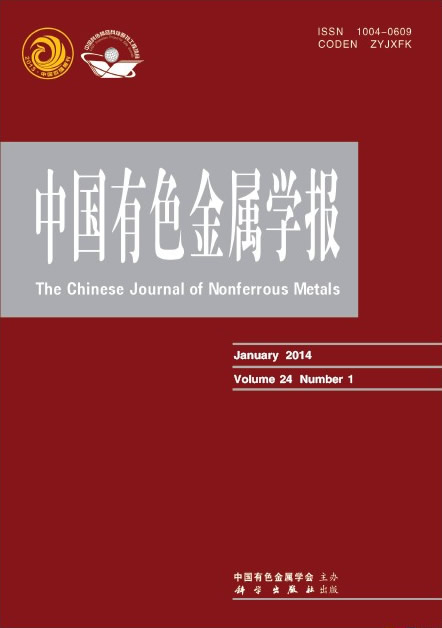## Transactions of Nonferrous Metals Society of China

 Vol. 25    No. 3    March 2015

Plotting and application of predominance area diagram of In-S-O system based on topological rules

1. Key Laboratory of Resources Chemistry of Nonferrous Metals, Ministry of Education,
School of Chemistry and Chemical Engineering, Central South University, Changsha 410083, China;
2. College of Mechanical Engineering, Hunan Institute of Engineering, Xiangtan 411101, China

Abstract:The mathematical topological rule was proposed to plot the predominance area diagram. Based on the phase rules, the components of In-S-O system were analyzed and the coexisting points of three condensed phases were determined. Combined with the topological rules and thermodynamic calculation, four relation diagrams between the coexisting points of three condensed phases, which were denoted as α, β stable plane-topological diagram and unstable plane-topological diagram, were plotted for the In-S-O system. The results show that α stable plane topological diagram is in accordance with the predominance area diagram of In-S-O system plotted by traditional methods, which indicates that the new method is feasible to plot the predominance area diagram of In-S-O system. Meanwhile, β unstable plane-topological diagram can be used to elucidate the indium production with the bath smelting process.

Key words: In-S-O system; predominance area diagram; topological rules; thermodynamic calculation; bath smeltingISSN 1004-0609
CN 43-1238/TG
CODEN: ZYJXFKISSN 1003-6326
CN 43-1239/TG
CODEN: TNMCEW

------------------------------------------------------------------------------------------